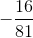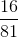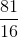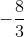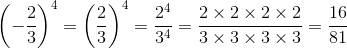ISEE Middle Level Math : How to multiply fractions

Example Questions

Example Question #11 : How To Multiply Fractions

Multiply the fractions: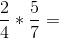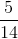Explanation:

To multiply fractions, multiply both numerators on top and both denominators on the bottom.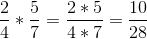Then, reduce to simplest form by removing any common factors: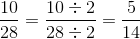Answer:Example Question #12 : Fractions

Solve: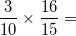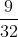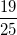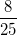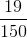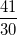Explanation: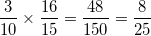Example Question #13 : Fractions

Solve for y: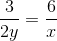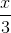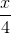Explanation:

In order to solve for y, cross multiplication must be used. Appyling cross multiplication, we get: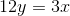Next, we divide each side by 12.

This results in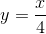Example Question #14 : Fractions

On Monday, Marsha saw a sweater that she wanted to buy. It was 15 dollars. She went back the next day and saw that it was only 10 dollars. Which of the following is a sale that the store could have been running, explaining the reduced price on the sweater?percent offoffpercent offoffoff

Explanation:

Given that the sweater's price was reduced by 5 dollars, this is a one third reduction because one third of 15 dollars is 5 dollars. 15 dollars minus 5 dollars is equal to 10 dollars.off

Example Question #12 : How To Multiply Fractions

One dollar is equal to about 116.76 Japanese yen. For how many yen should a tourist to Japan be able to exchange $3,000 at that rate (nearest whole yen)? Possible Answers: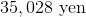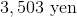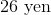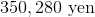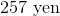Correct answer:Explanation: One dollar is equal to 116.76 yen, so multiply$3,000 by this conversion rate to get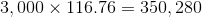yen.

Example Question #12 : How To Multiply Fractions

Evaluate: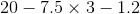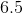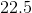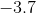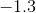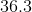Explanation:

By the order of operations, carry out the multiplication first, then the leftmost subtraction, then the rightmost subtraction: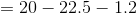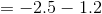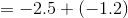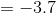Example Question #351 : Numbers And Operations

Raiseto the fifth power.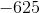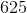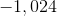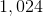cannot be raised to the fifth power.Explanation:

To raise a negative number to an odd-numbered power, raise its absolute value to that power, then make the sign negative: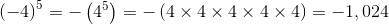Example Question #352 : Numbers And Operations

Raiseto the fourth power.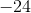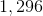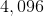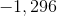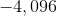Explanation:

To raise a negative number to an even-numbered power, raise its absolute value to that power: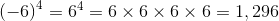Example Question #19 : How To Multiply Fractions

Evaluate: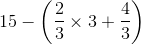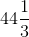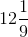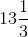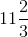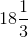Explanation:

By the order of operations, carry out the operations in parentheses first; since there is a multiplication and an addition present, carry them out in that order. Finally, carry out the subtraction: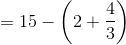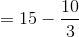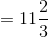Example Question #20 : How To Multiply Fractions

Raise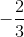to the fourth power.